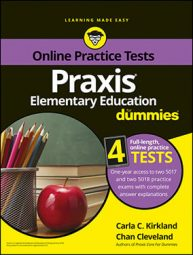##### Praxis Elementary Education For Dummies with Online Practice TestsWhen you run into problems on the Praxis Elementary Education exam that involve using the four major math operations—adding, subtracting, multiplying, and dividing—you can use PEMDAS to solve them.

When multiple operations are used in the working of a math problem, there is an order in which the operations must take place. False orders can cause false answers, but the true order of operations, which is called the order of operations, results in true answers when the calculations involved are correct. The order of operations is often represented by the acronym PEMDAS, which represents the following order: parentheses and other grouping symbols (such as brackets and fraction bars), exponents, multiplication and division from left to right, addition and subtraction from left to right. The acronym PEMDAS has a long history of successfully helping students remember the order of operations.

## Practice question

1. What is the value of the following expression?

A. 0 B. 24 C. 35 D. 4

1. The correct answer is Choice (D). Determining the value of the expression involves performing multiple operations, and doing that requires following the order of operations. That order is represented by the acronym PEMDAS, which stands for "parentheses and other grouping symbols, exponents, multiplication and division from left to right, addition and subtraction from left to right."

The other choices can result from following false orders of operations.